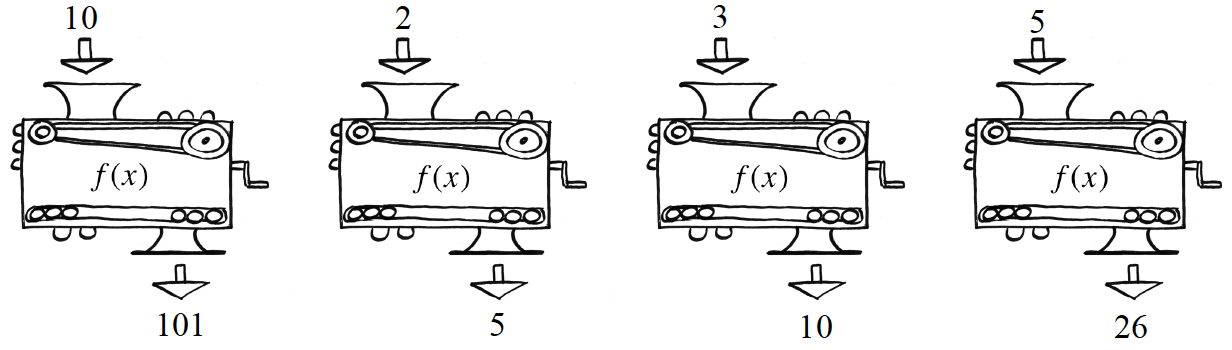### Home > A2C > Chapter Ch4 > Lesson 4.3.2 > Problem4-152

4-152.

Remember function machines? Each of the following pictures shows how the same machine changes the given $x$-value into a corresponding $f (x)$ value. Find the equation for this machine.Copy and complete the table for the function.

 $x$ $10\ \ \ \ 2\ \ \ \ 3$ $f\left(x\right)$ $101\ \ \ 5$

$f(x)=x^2+1$